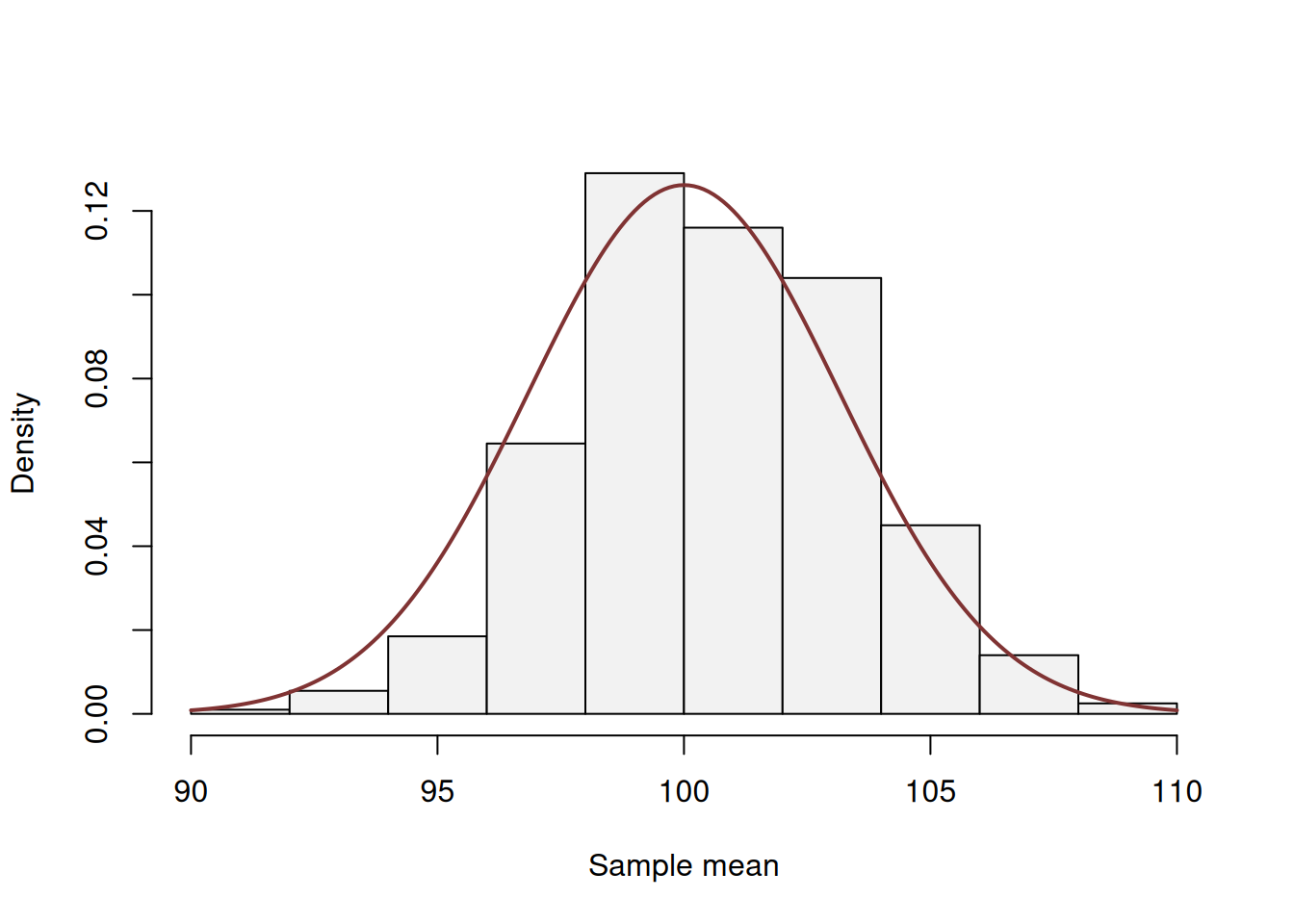This book is in Open Review. I want your feedback to make the book better for you and other readers. To add your annotation, select some text and then click the on the pop-up menu. To see the annotations of others, click the button in the upper right hand corner of the page

## 6.2 Central Limit Theorem

As we have already seen on Figure 6.2, the sample mean is not exactly equal to the population mean even when the sample size is very large (thousands of observations). There is always some sort of variability around the population mean. In order to understand how this variability looks like, we could conduct a simple experiment. We could take a random sample of, for instance, 1000 observations several times and record each of the obtained means. We then can see how the variable will be distributed to see if there are any patterns in the behaviour of the estimator:

nIterations <- 1000
yMean <- vector("numeric",nIterations)
for(i in 1:nIterations){
yMean[i] <- mean(sample(y,1000))
}
hist(yMean, xlab="Sample mean", main="")Figure 6.3: Histogram of the mean of the variable y.

There is a theorem that says that the distribution of mean in the experiment above will follow normal distribution under several conditions (discussed later in this section). It is called Central Limit Theorem (CLT) and very roughly it says that when independent random variables are added, their normalised sum will asymptotically follow normal distribution, even if the original variables do not follow it. Note that this is the theorem about what happens with the estimate (sum in this case), not with individual observations. This means that the error term might follow, for example, Inverse Gaussian distribution, but the estimate of its mean (under some conditions) will follow normal distribution. There are different versions of this theorem, built with different assumptions with respect to the random variable and the estimation procedure, but we do not dicuss these details in this textbook.

In order for CLT to hold, the following important assumptions need to be satisfied:

1. The true value of parameter is not near the bound. e.g. if the variable follows uniform distribution on (0, $$a$$) and we want to estimate $$a$$, then its distribution will not be Normal (because in this case the true value is always approached from below). This assumption is important in our context, because ETS and ARIMA typically have restrictions on their parameters.
2. The random variables are identically independent distributed (i.i.d.). If they are not, then their average might not follow normal distribution (in some conditions it still might).
3. The mean and variance of the distribution are finite. This might seem as a weird issue, but some distributions do not have finite moments, so the CLT will not hold if a variable follows them, just because the sample mean will be all over the plane due to randomness and will not converge to the “true” value. Cauchy distribution is one of such examples.

If these assumptions hold, then CLT will work for the estimate of a parameter, no matter what the distribution of the random variable is. This becomes especially useful, when we want to test a hypothesis or construct a confidence interval for an estimate of a parameter.